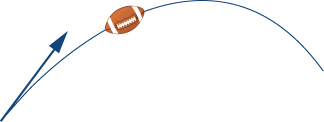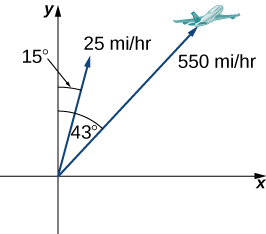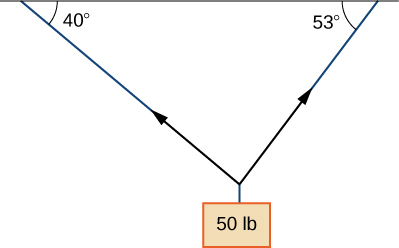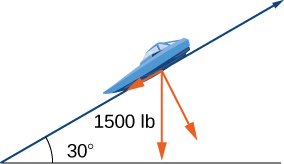$$\newcommand{\id}{\mathrm{id}}$$ $$\newcommand{\Span}{\mathrm{span}}$$ $$\newcommand{\kernel}{\mathrm{null}\,}$$ $$\newcommand{\range}{\mathrm{range}\,}$$ $$\newcommand{\RealPart}{\mathrm{Re}}$$ $$\newcommand{\ImaginaryPart}{\mathrm{Im}}$$ $$\newcommand{\Argument}{\mathrm{Arg}}$$ $$\newcommand{\norm}{\| #1 \|}$$ $$\newcommand{\inner}{\langle #1, #2 \rangle}$$ $$\newcommand{\Span}{\mathrm{span}}$$

# 11.1E: Exercises for Vectors in the Plane

$$\newcommand{\vecs}{\overset { \rightharpoonup} {\mathbf{#1}} }$$ $$\newcommand{\vecd}{\overset{-\!-\!\rightharpoonup}{\vphantom{a}\smash {#1}}}$$$$\newcommand{\id}{\mathrm{id}}$$ $$\newcommand{\Span}{\mathrm{span}}$$ $$\newcommand{\kernel}{\mathrm{null}\,}$$ $$\newcommand{\range}{\mathrm{range}\,}$$ $$\newcommand{\RealPart}{\mathrm{Re}}$$ $$\newcommand{\ImaginaryPart}{\mathrm{Im}}$$ $$\newcommand{\Argument}{\mathrm{Arg}}$$ $$\newcommand{\norm}{\| #1 \|}$$ $$\newcommand{\inner}{\langle #1, #2 \rangle}$$ $$\newcommand{\Span}{\mathrm{span}}$$ $$\newcommand{\id}{\mathrm{id}}$$ $$\newcommand{\Span}{\mathrm{span}}$$ $$\newcommand{\kernel}{\mathrm{null}\,}$$ $$\newcommand{\range}{\mathrm{range}\,}$$ $$\newcommand{\RealPart}{\mathrm{Re}}$$ $$\newcommand{\ImaginaryPart}{\mathrm{Im}}$$ $$\newcommand{\Argument}{\mathrm{Arg}}$$ $$\newcommand{\norm}{\| #1 \|}$$ $$\newcommand{\inner}{\langle #1, #2 \rangle}$$ $$\newcommand{\Span}{\mathrm{span}}$$

For exercises 1-10, consider points $$P(−1,3), Q(1,5),$$ and $$R(−3,7)$$. Determine the requested vectors and express each of them

$$a.$$ in component form and

$$b.$$ by using standard unit vectors.

1) $${\overset{-\!-\!\rightharpoonup}{\vphantom{a}\smash{PQ}}}$$

$$a. \vec{PQ}=⟨2,2⟩ \quad b. \vec{PQ}=2\hat{\mathbf i}+2\hat{\mathbf j}$$

2) $$\vec{PR}$$

3) $$\vec{QP}$$

$$a. \vec{QP}=⟨−2,−2⟩ \quad b. \vec{QP}=−2\hat{\mathbf i}−2\hat{\mathbf j}$$

4) $$\vec{RP}$$

5) $$\vec{PQ}+\vec{PR}$$

$$a. \vec{PQ}+\vec{PR}=⟨0,6⟩ \quad b. \vec{PQ}+\vec{PR}=6\hat{\mathbf j}$$

6) $$\vec{PQ}−\vec{PR}$$

7) $$2\vec{PQ}−2\vec{PR}$$

$$a. 2\vec{PQ}→−2\vec{PR}=⟨8,−4⟩ \quad b. 2\vec{PQ}−2\vec{PR}=8\hat{\mathbf i}−4\hat{\mathbf j}$$

8) $$2\vec{PQ}+\frac{1}{2}\vec{PR}$$

9) The unit vector in the direction of $$\vec{PQ}$$

$$a. ⟨\frac{1}{\sqrt{2}},\frac{1}{\sqrt{2}}⟩ \quad b. \frac{1}{\sqrt{2}}\hat{\mathbf i}+\frac{1}{\sqrt{2}}\hat{\mathbf j}$$

10) The unit vector in the direction of $$\vec{PR}$$

11) A vector $${\overset{\scriptstyle\rightharpoonup}{\mathbf v}}$$ has initial point $$(−1,−3)$$ and terminal point $$(2,1)$$. Find the unit vector in the direction of $${\overset{\scriptstyle\rightharpoonup}{\mathbf v}}$$. Express the answer in component form.

$$⟨\frac{3}{5},\frac{4}{5}⟩$$

12) A vector $$\vec{\mathbf v}$$ has initial point $$(−2,5)$$ and terminal point $$(3,−1)$$. Find the unit vector in the direction of $$\vec{\mathbf v}$$. Express the answer in component form.

13) The vector $$\vec{\mathbf v}$$ has initial point $$P(1,0)$$ and terminal point $$Q$$ that is on the y-axis and above the initial point. Find the coordinates of terminal point $$Q$$ such that the magnitude of the vector $$\vec{\mathbf v}$$ is $$\sqrt{5}$$.

$$Q(0,2)$$

14) The vector $$\vec{\mathbf v}$$ has initial point $$P(1,1)$$ and terminal point $$Q$$ that is on the x-axis and left of the initial point. Find the coordinates of terminal point $$Q$$ such that the magnitude of the vector $$\vec{\mathbf v}$$ is $$\sqrt{10}$$.

For exercises 15 and 16, use the given vectors $$\vec{\mathbf a}$$ and $$\vec{\mathbf b}$$.

a. Determine the vector sum $$\vec{\mathbf a}+\vec{\mathbf b}$$ and express it in both the component form and by using the standard unit vectors.

b. Find the vector difference $$\vec{\mathbf a}−\vec{\mathbf b}$$ and express it in both the component form and by using the standard unit vectors.

c. Verify that the vectors $$\vec{\mathbf a}, \, \vec{\mathbf b},$$ and $$\vec{\mathbf a}+\vec{\mathbf b}$$, and, respectively, $$\vec{\mathbf a}, \, \vec{\mathbf b}$$, and $$\vec{\mathbf a}−\vec{\mathbf b}$$ satisfy the triangle inequality.

d. Determine the vectors $$2\vec{\mathbf a}, −\vec{\mathbf b},$$ and $$2\vec{\mathbf a}−\vec{\mathbf b}.$$ Express the vectors in both the component form and by using standard unit vectors.

15) $$\vec{\mathbf a}=2\hat{\mathbf i}+\hat{\mathbf j}, \vec{\mathbf b}=\hat{\mathbf i}+3\hat{\mathbf j}$$

$$a.\, \vec{\mathbf a}+\vec{\mathbf b}=⟨3,4⟩, \quad \vec{\mathbf a}+\vec{\mathbf b}=3\hat{\mathbf i}+4\hat{\mathbf j}$$
$$b.\, \vec{\mathbf a}−\vec{\mathbf b}=⟨1,−2⟩, \quad \vec{\mathbf a}−\vec{\mathbf b}=\hat{\mathbf i}−2\hat{\mathbf j}$$
$$c.$$ Answers will vary
$$d.\, 2\vec{\mathbf a}=⟨4,2⟩, \quad 2\vec{\mathbf a}=4\hat{\mathbf i}+2\hat{\mathbf j}, \quad −\vec{\mathbf b}=⟨−1,−3⟩, \quad −\vec{\mathbf b}=−\hat{\mathbf i}−3\hat{\mathbf j}, \quad 2\vec{\mathbf a}−\vec{\mathbf b}=⟨3,−1⟩, \quad 2\vec{\mathbf a}−\vec{\mathbf b}=3\hat{\mathbf i}−\hat{\mathbf j}$$

16) $$\vec{\mathbf a}=2\hat{\mathbf i}, \vec{\mathbf b}=−2\hat{\mathbf i}+2\hat{\mathbf j}$$

17) Let $$\vec{\mathbf a}$$ be a standard-position vector with terminal point $$(−2,−4)$$. Let $$\vec{\mathbf b}$$ be a vector with initial point $$(1,2)$$ and terminal point $$(−1,4)$$. Find the magnitude of vector $$−3\vec{\mathbf a}+\vec{\mathbf b}−4\hat{\mathbf i}+\hat{\mathbf j}.$$

$$15$$

18) Let $$\vec{\mathbf a}$$ be a standard-position vector with terminal point at $$(2,5)$$. Let $$\vec{\mathbf b}$$ be a vector with initial point $$(−1,3)$$ and terminal point $$(1,0)$$. Find the magnitude of vector $$\vec{\mathbf a}−3\vec{\mathbf b}+14\hat{\mathbf i}−14\hat{\mathbf j}.$$

19) Let $$\vec{\mathbf u}$$ and $$\vec{\mathbf v}$$ be two nonzero vectors that are nonequivalent. Consider the vectors $$\vec{\mathbf a}=4\vec{\mathbf u}+5\vec{\mathbf v}$$ and $$\vec{\mathbf b}=\vec{\mathbf u}+2\vec{\mathbf v}$$ defined in terms of $$\vec{\mathbf u}$$ and $$\vec{\mathbf v}$$. Find the scalar $$λ$$ such that vectors $$\vec{\mathbf a}+λ\vec{\mathbf b}$$ and $$\vec{\mathbf u}−\vec{\mathbf v}$$ are equivalent.

$$λ=−3$$

20) Let $$\vec{\mathbf u}$$ and $$\vec{\mathbf v}$$ be two nonzero vectors that are nonequivalent. Consider the vectors $$\vec{\mathbf a}=2\vec{\mathbf u}−4\vec{\mathbf v}$$ and $$\vec{\mathbf b}=3\vec{\mathbf u}−7\vec{\mathbf v}$$ defined in terms of $$\vec{\mathbf u}$$ and $$\vec{\mathbf v}$$. Find the scalars $$α$$ and $$β$$ such that vectors $$α\vec{\mathbf a}+β\vec{\mathbf b}$$ and $$\vec{\mathbf u}−\vec{\mathbf v}$$ are equivalent.

21) Consider the vector $$\vec{\mathbf a}(t)=⟨\cos t, \sin t⟩$$ with components that depend on a real number $$t$$. As the number $$t$$ varies, the components of $$\vec{\mathbf a}(t)$$ change as well, depending on the functions that define them.

a. Write the vectors $$\vec{\mathbf a}(0)$$ and $$\vec{\mathbf a}(π)$$ in component form.

b. Show that the magnitude $$∥\vec{\mathbf a}(t)∥$$ of vector $$\vec{\mathbf a}(t)$$ remains constant for any real number $$t$$.

c. As $$t$$ varies, show that the terminal point of vector $$\vec{\mathbf a}(t)$$ describes a circle centered at the origin of radius $$1$$.

$$a.\, \vec{\mathbf a}(0)=⟨1,0⟩, \quad \vec{\mathbf a}(π)=⟨−1,0⟩$$
$$b.$$ Answers may vary
$$c.$$ Answers may vary

22) Consider vector $$\vec{\mathbf a}(x)=⟨x,\sqrt{1−x^2}⟩$$ with components that depend on a real number $$x∈[−1,1]$$. As the number $$x$$ varies, the components of $$\vec{\mathbf a}(x)$$ change as well, depending on the functions that define them.

a. Write the vectors $$\vec{\mathbf a}(0)$$ and $$\vec{\mathbf a}(1)$$ in component form.

b. Show that the magnitude $$∥\vec{\mathbf a}(x)∥$$ of vector $$\vec{\mathbf a}(x)$$ remains constant for any real number $$x$$

c. As $$x$$ varies, show that the terminal point of vector $$\vec{\mathbf a}(x)$$ describes a circle centered at the origin of radius $$1$$.

23) Show that vectors $$\vec{\mathbf a}(t)=⟨\cos t, \sin t⟩$$ and $$\vec{\mathbf a}(x)=⟨x,\sqrt{1−x^2}⟩$$ are equivalent for $$x=r$$ and $$t=2kπ$$, where $$k$$ is an integer.

24) Show that vectors $$\vec{\mathbf a}(t)=⟨\cos t, \sin t⟩$$ and $$\vec{\mathbf a}(x)=⟨x,\sqrt{1−x^2}⟩$$ are opposite for $$x=r$$ and $$t=π+2kπ$$, where $$k$$ is an integer.

For exercises 25-28, find a vector $$\vec{\mathbf v}$$ with the given magnitude and in the same direction as the vector $$\vec{\mathbf u}$$.

25) $$\|\vec{\mathbf v}\|=7, \quad \vec{\mathbf u}=⟨3,4⟩$$

$$\vec{\mathbf v}=⟨\frac{21}{5},\frac{28}{5}⟩$$

26) $$‖\vec{\mathbf v}‖=3,\quad \vec{\mathbf u}=⟨−2,5⟩$$

27) $$‖\vec{\mathbf v}‖=7,\quad \vec{\mathbf u}=⟨3,−5⟩$$

$$\vec{\mathbf v}=⟨\frac{21\sqrt{34}}{34},−\frac{35\sqrt{34}}{34}⟩$$

28) $$‖\vec{\mathbf v}‖=10,\quad \vec{\mathbf u}=⟨2,−1⟩$$

For exercises 29-34, find the component form of vector $$\vec{\mathbf u}$$, given its magnitude and the angle the vector makes with the positive x-axis. Give exact answers when possible.

29) $$‖\vec{\mathbf u}‖=2, θ=30°$$

$$\vec{\mathbf u}=⟨\sqrt{3},1⟩$$

30) $$‖\vec{\mathbf u}‖=6, θ=60°$$

31) $$‖\vec{\mathbf u}‖=5, θ=\frac{π}{2}$$

$$\vec{\mathbf u}=⟨0,5⟩$$

32) $$‖\vec{\mathbf u}‖=8, θ=π$$

33) $$‖\vec{\mathbf u}‖=10, θ=\frac{5π}{6}$$

$$\vec{\mathbf u}=⟨−5\sqrt{3},5⟩$$

34) $$‖\vec{\mathbf u}‖=50, θ=\frac{3π}{4}$$

For exercises 35 and 36, vector $$\vec{\mathbf u}$$ is given. Find the angle $$θ∈[0,2π)$$ that vector $$\vec{\mathbf u}$$ makes with the positive direction of the x-axis, in a counter-clockwise direction.

35) $$\vec{\mathbf u}=5\sqrt{2}\hat{\mathbf i}−5\sqrt{2}\hat{\mathbf j}$$

$$θ=\frac{7π}{4}$$

36) $$\vec{\mathbf u}=−\sqrt{3}\hat{\mathbf i}−\hat{\mathbf j}$$

37) Let $$\vec{\mathbf a}=⟨a_1,a_2⟩, \vec{\mathbf b}=⟨b_1,b_2⟩$$, and $$\vec{\mathbf c}=⟨c_1,c_2⟩$$ be three nonzero vectors. If $$a_1b_2−a_2b_1≠0$$, then show there are two scalars, $$α$$ and $$β$$, such that $$\vec{\mathbf c}=α\vec{\mathbf a}+β\vec{\mathbf b}.$$

38) Consider vectors $$\vec{\mathbf a}=⟨2,−4⟩, \vec{\mathbf b}=⟨−1,2⟩,$$ and $$\vec{\mathbf c}=\vec{\mathbf 0}$$ Determine the scalars $$α$$ and $$β$$ such that $$\vec{\mathbf c}=α\vec{\mathbf a}+β\vec{\mathbf b}$$.

39) Let $$P(x_0,f(x_0))$$ be a fixed point on the graph of the differential function $$f$$ with a domain that is the set of real numbers.

a. Determine the real number $$z_0$$ such that point $$Q(x_0+1,z_0)$$ is situated on the line tangent to the graph of $$f$$ at point $$P$$.

b. Determine the unit vector $$\vec{\mathbf u}$$ with initial point $$P$$ and terminal point $$Q$$.

$$a. \quad z_0=f(x_0)+f′(x_0); \quad b. \quad \vec{\mathbf u}=\frac{1}{\sqrt{1+[f′(x_0)]^2}}⟨1,f′(x_0)⟩$$

40) Consider the function $$f(x)=x^4,$$ where $$x∈R$$.

a. Determine the real number $$z_0$$ such that point $$Q(2,z_0)$$ s situated on the line tangent to the graph of $$f$$ at point $$P(1,1)$$.

b. Determine the unit vector $$\vec{\mathbf u}$$ with initial point $$P$$ and terminal point $$Q$$.

41) Consider $$f$$ and $$g$$ two functions defined on the same set of real numbers $$D$$. Let $$\vec{\mathbf a}=⟨x,f(x)⟩$$ and $$\vec{\mathbf b}=⟨x,g(x)⟩$$ be two vectors that describe the graphs of the functions, where $$x∈D$$. Show that if the graphs of the functions $$f$$ and $$g$$ do not intersect, then the vectors $$\vec{\mathbf a}$$ and $$\vec{\mathbf b}$$ are not equivalent.

42) Find $$x∈R$$ such that vectors $$\vec{\mathbf a}=⟨x, \sin x⟩$$ and $$\vec{\mathbf b}=⟨x, \cos x⟩$$ are equivalent.

43) Calculate the coordinates of point $$D$$ such that $$ABCD$$ is a parallelogram, with $$A(1,1), B(2,4)$$, and $$C(7,4)$$.

$$D(6,1)$$

44) Consider the points $$A(2,1), B(10,6), C(13,4)$$, and $$D(16,−2)$$. Determine the component form of vector $$\vec{AD}$$.

45) The speed of an object is the magnitude of its related velocity vector. A football thrown by a quarterback has an initial speed of $$70$$ mph and an angle of elevation of $$30°$$. Determine the velocity vector in mph and express it in component form. (Round to two decimal places.)$$⟨60.62,35⟩$$

46) A baseball player throws a baseball at an angle of $$30°$$ with the horizontal. If the initial speed of the ball is $$100$$ mph, find the horizontal and vertical components of the initial velocity vector of the baseball. (Round to two decimal places.)

47) A bullet is fired with an initial velocity of $$1500$$ ft/sec at an angle of $$60°$$ with the horizontal. Find the horizontal and vertical components of the velocity vector of the bullet. (Round to two decimal places.)The horizontal and vertical components are $$750$$ ft/sec and $$1299.04$$ ft/sec, respectively.

48) [T] A 65-kg sprinter exerts a force of $$798$$ N at a $$19°$$ angle with respect to the ground on the starting block at the instant a race begins. Find the horizontal component of the force. (Round to two decimal places.)

49) [T] Two forces, a horizontal force of $$45$$ lb and another of $$52$$ lb, act on the same object. The angle between these forces is $$25°$$. Find the magnitude and direction angle from the positive x-axis of the resultant force that acts on the object. (Round to two decimal places.)The magnitude of resultant force is $$94.71$$ lb; the direction angle is $$13.42°$$.

50) [T] Two forces, a vertical force of $$26$$ lb and another of $$45$$ lb, act on the same object. The angle between these forces is $$55°$$. Find the magnitude and direction angle from the positive x-axis of the resultant force that acts on the object. (Round to two decimal places.)

51) [T] Three forces act on object. Two of the forces have the magnitudes $$58$$ N and $$27$$ N, and make angles $$53°$$ and $$152°$$, respectively, with the positive x-axis. Find the magnitude and the direction angle from the positive x-axis of the third force such that the resultant force acting on the object is zero. (Round to two decimal places.)

The magnitude of the third vector is $$60.03$$N; the direction angle is $$259.38°$$.

52) Three forces with magnitudes 80 lb, 120 lb, and 60 lb act on an object at angles of $$45°, 60°$$ and $$30°$$, respectively, with the positive x-axis. Find the magnitude and direction angle from the positive x-axis of the resultant force. (Round to two decimal places.)53) [T] An airplane is flying in the direction of $$43°$$ east of north (also abbreviated as $$N43E$$ at a speed of $$550$$ mph. A wind with speed $$25$$ mph comes from the southwest at a bearing of $$N15E$$. What are the ground speed and new direction of the airplane?The new ground speed of the airplane is $$572.19$$ mph; the new direction is $$N41.82E.$$

54) [T] A boat is traveling in the water at $$30$$ mph in a direction of $$N20E$$ (that is, $$20°$$ east of north). A strong current is moving at $$15$$ mph in a direction of $$N45E$$. What are the new speed and direction of the boat?55) [T] A 50-lb weight is hung by a cable so that the two portions of the cable make angles of $$40°$$ and $$53°$$, respectively, with the horizontal. Find the magnitudes of the forces of tension $$\vec{\mathbf T_1}$$ and $$\vec{\mathbf T_2}$$ in the cables if the resultant force acting on the object is zero. (Round to two decimal places.)$$\|\vec{\mathbf T_1}\|=30.13 \, lb, \quad \|\vec{\mathbf T_2}\|=38.35 \, lb$$

56) [T] A 62-lb weight hangs from a rope that makes the angles of $$29°$$ and $$61°$$, respectively, with the horizontal. Find the magnitudes of the forces of tension $$\vec{\mathbf T_1}$$ and $$\vec{\mathbf T_2}$$ in the cables if the resultant force acting on the object is zero. (Round to two decimal places.)

57) [T] A 1500-lb boat is parked on a ramp that makes an angle of $$30°$$ with the horizontal. The boat’s weight vector points downward and is a sum of two vectors: a horizontal vector $$\vec{\mathbf v_1}$$ that is parallel to the ramp and a vertical vector $$\vec{\mathbf v_2}$$ that is perpendicular to the inclined surface. The magnitudes of vectors $$\vec{\mathbf v_1}$$ and $$\vec{\mathbf v_2}$$ are the horizontal and vertical component, respectively, of the boat’s weight vector. Find the magnitudes of $$\vec{\mathbf v_1}$$ and $$\vec{\mathbf v_2}$$. (Round to the nearest integer.)$$\|\vec{\mathbf v_1}\|=750 \, lb, \quad \|\vec{\mathbf v_2}\|=1299 \, lb$$

58) [T] An 85-lb box is at rest on a $$26°$$ incline. Determine the magnitude of the force parallel to the incline necessary to keep the box from sliding. (Round to the nearest integer.)

59) A guy-wire supports a pole that is $$75$$ ft high. One end of the wire is attached to the top of the pole and the other end is anchored to the ground $$50$$ ft from the base of the pole. Determine the horizontal and vertical components of the force of tension in the wire if its magnitude is $$50$$ lb. (Round to the nearest integer.)The two horizontal and vertical components of the force of tension are $$28$$ lb and $$42$$ lb, respectively.
60) A telephone pole guy-wire has an angle of elevation of $$35°$$ with respect to the ground. The force of tension in the guy-wire is $$120$$ lb. Find the horizontal and vertical components of the force of tension. (Round to the nearest integer.)